#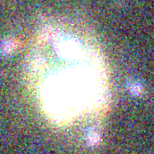UIT008 (J013245.41+303858.3 )

## Target Notes### Classification: Of/late-WN

(Candidate LBV) Two LGGS stars >2 fainter and fifteen LGGS stars >3 magnitudes fainter within 5 arcseconds. But nothing else within 2 arcseconds.

## Plots of Brightness and Color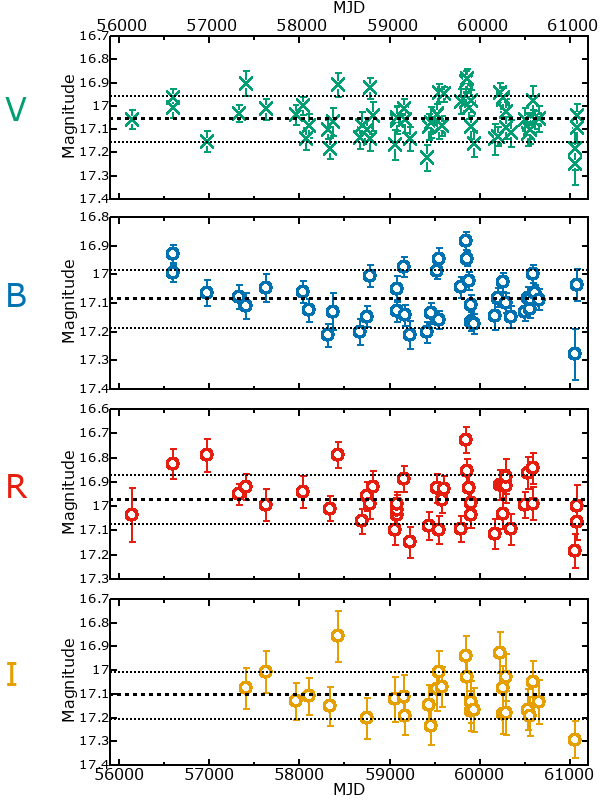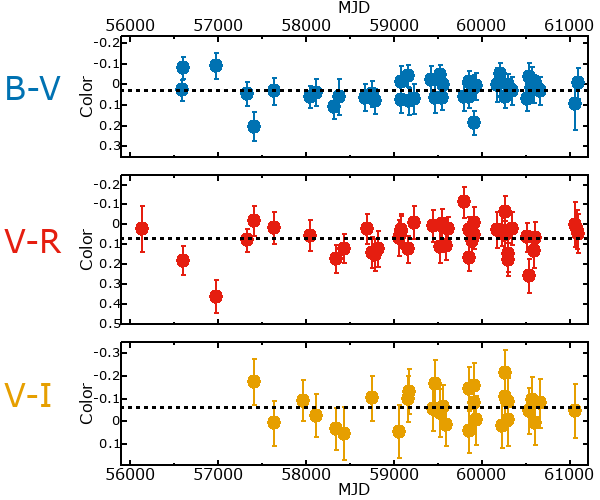## Variability

From the data available in this survey, it is not possible to tell with certainty if this star is variable.

### Correlation factors between bands

If the brightness variation is true variability then there should be a correlation between brightness changes recorded in different filter bands. The following table are the R-squared linear correlation factors calculated for the pairs of bands. The number in parentheses next to the R-squared value is the number of elements used to calculate the correlation factor. The number in square brackets next to the band is the range of magnitudes measured.

V [0.40 mag]B [0.54 mag]R [0.53 mag]
B [0.40 mag]0.06 (19)
R [0.53 mag]0.16 (17)0.61 (11)
I [0.34 mag]0.25 (9)0.36 (6)0.55 (6)

### Magnitude-Magnitude Plots

The plots below are provided to check the values in the table above and also to show any non-linear correlations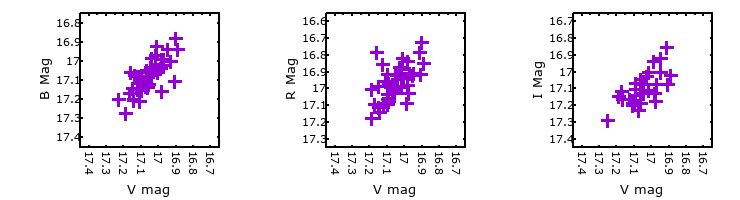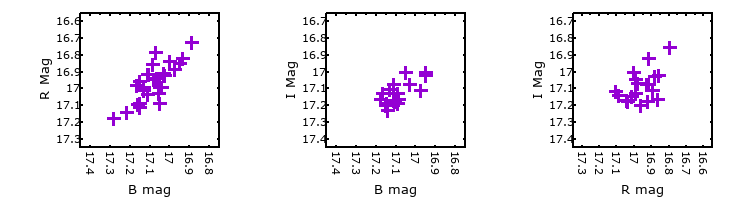### I & R Statistics

Welch & Stetson (1993, AJ, 105, 1813) present a method for varible star detection in CCD images from automated surveys. Data from two bands are compared and two statistics are calculated: R and I.

The I-statistic has an expectation value of zero for stars that are NOT variable. A larger non-zero I-value indicates a higher likelihood that the star is variable. The R statistic indicates how the color of the star changes as the variable grows brighter with R = 1.0 being no change in color.

R and I values have only been calculated if there are more than four (4) matched measurements of brightness in each band.
V
(I|R)
B
(I|R)
R
(I|R)
B
(I|R)
2.26 | 1.21
R
(I|R)
2.09 | 1.142.08 | 1.05
I
(I|R)
1.03 | 1.380.77 | 2.361.68 | 1.05